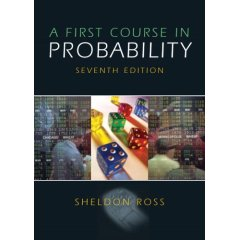# A First Course In Probability: Seventh Edition

## by Sheldon Ross.Introduction
This book is one of the best probability books written. The explanations are very clear while at the same time the material covered is more advanced and interesting that what is commonly presented in undergraduate textbooks on probability. The problems are a real treasure, in that they are very interesting and challenging. Earlier I was working with a few students who are taking a MIT course 18.440 using this book. As they worked through the book, I worked the problems also and wrote up my solutions.

When I made this write-up, I was working with the seventh edition of this book. I don't think there are many differences between later/earlier editions, however.

Update (2013-07-21): After about 10 years of giving this material away for free (in PDF format) I've decided to try to sell copies of it on Amazon. Please look here for the most recent version of the solution manual.

Rather than use a calculator or do any numerical calculations by hand, I used Matlab in many cases. You can any scripts or functions (often very short) created for a problem in the links below.

Code For Various Problems:
Chapter 1 (Combinatorial Analysis):
Chapter 2 (Axioms of Probability):
Chapter 3 (Conditional Probability and Independence):
Chapter 4 (Random Variables):
Chapter 5 (Continuous Random Variables):
Chapter 6 (Jointly Distributed Random Variables):
Chapter 7 (Properties of Expectation):
Chapter 8 (Limit Theorems):
Chapter 9 (Additional Topics in Probability):

John Weatherwax
Last modified: Sun Aug 25 08:23:35 EDT 2013